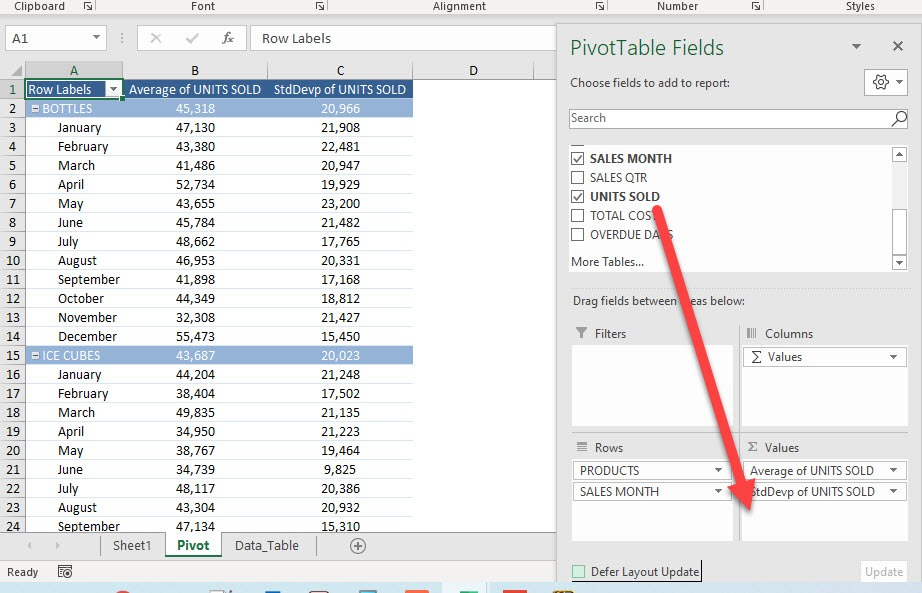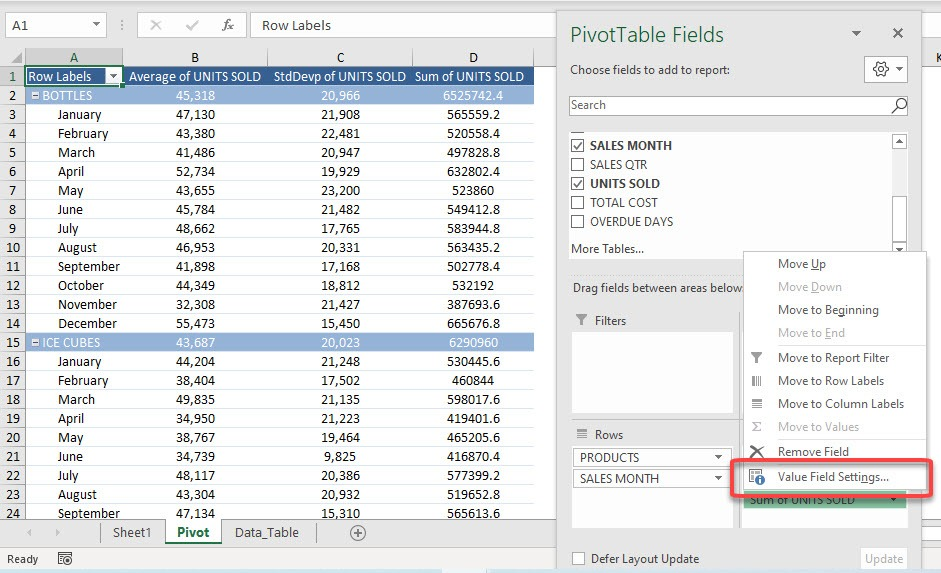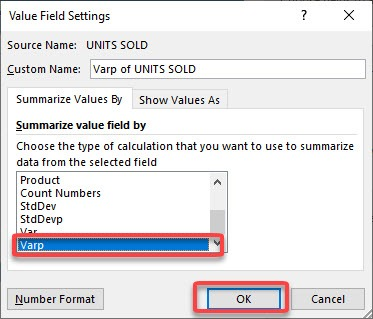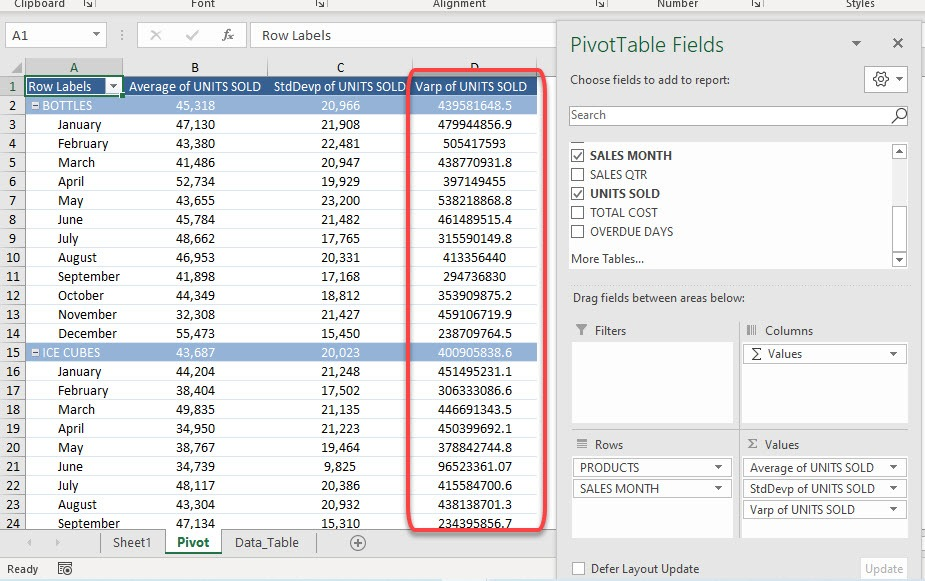It is very easy to use a Pivot Table to summarize data for you by giving the sum, count, or average. But did you know it can give you the standard deviation and variance as well? This is crucial if you want to understand how volatile your data is!

Let us explore how to get Varp in Pivot Table, this shows the variability from the average or mean value of the group.

Exercise Workbook:

STEP 1: Here is our Pivot Table. Drag UNITS SOLD to the Values AreaSTEP 2: This will default to Sum of UNITS SOLD. Let us change that by clicking on the arrow and selecting Value Field SettingsSTEP 3: Select Varp and click OK.

We will use the Varp function as we have the complete data (population) used in the calculation. When only a portion of the data is used, then Var should be used instead.Did you know that the square root of the variance will give you the standard deviation value? Click here to learn how!